# 双功能梯度纳米梁系统振动分析的辛方法 ∗

(大连理工大学 国际计算力学中心 工程力学系;工业装备结构分析国家重点实验室(大连理工大学)， 辽宁 大连 116024)

Hamilton体系； 辛方法； 双功能梯度纳米梁系统； 自由振动； 解析解

## 1 非局部场理论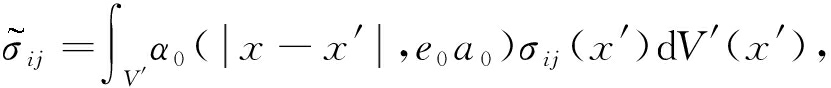x V

(1)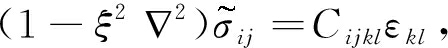(2)

## 2 功能梯度材料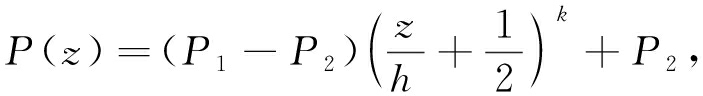(3)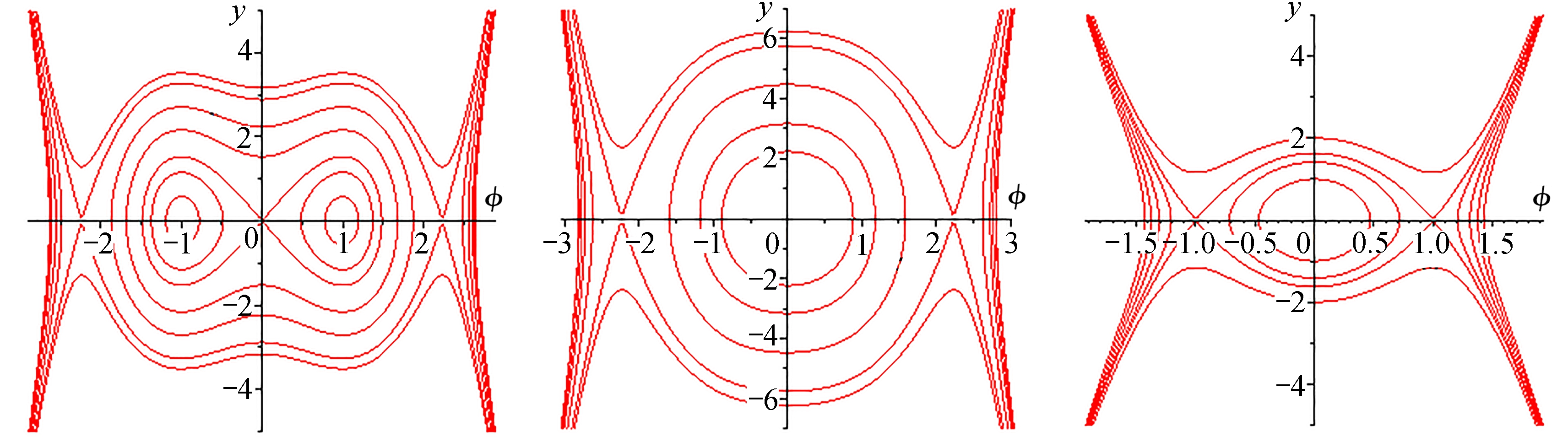Fig. 1 A functionally graded double-nanobeam system embedded in viscoelastic medium

## 3 基 本 方 程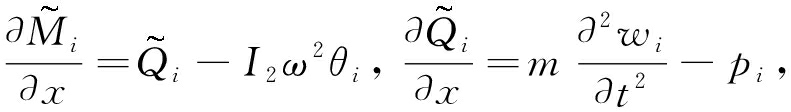(4)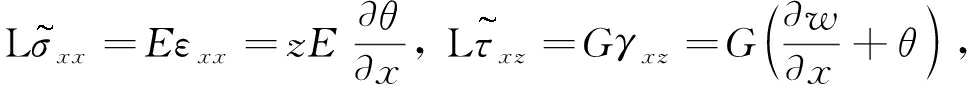(5)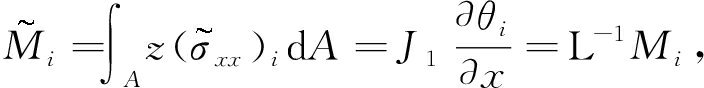(6a)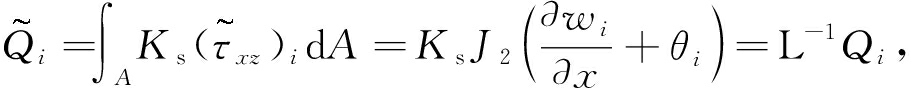(6b)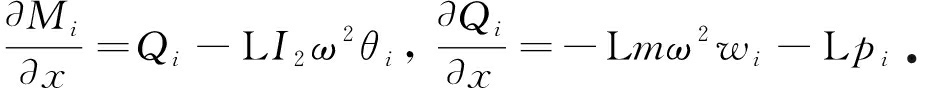(7)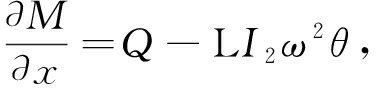(8a)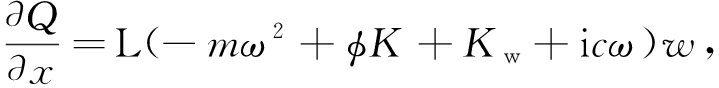(8b)

① S: w =0, M =0, when x =0, a

(9a)

② C: w =0, θ =0, when x =0, a

(9b)

③ F: M =0, Q =0, when x =0, a

(9c)

## 4 Hamilton体系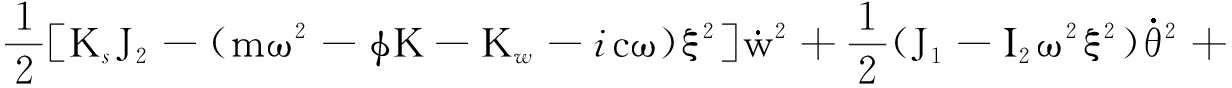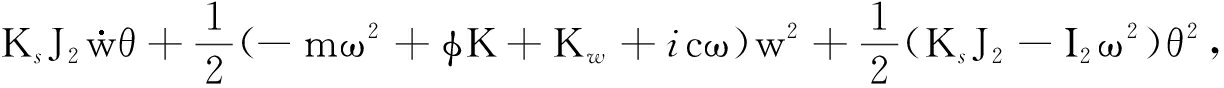(10)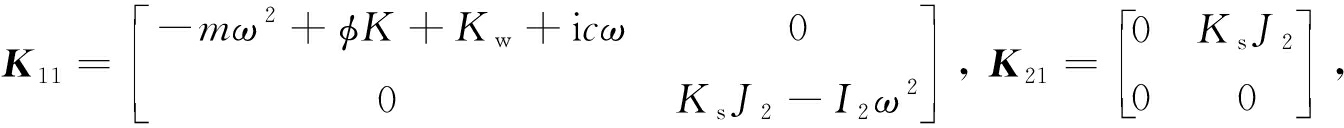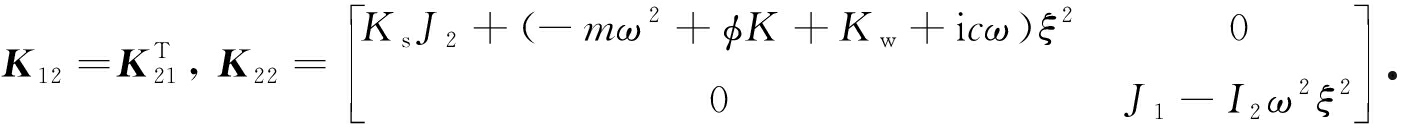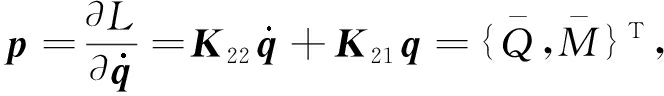(11)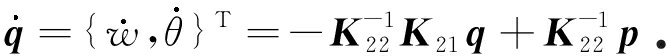(12)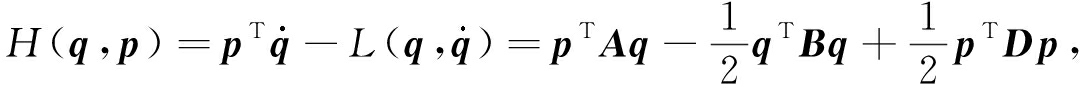(13)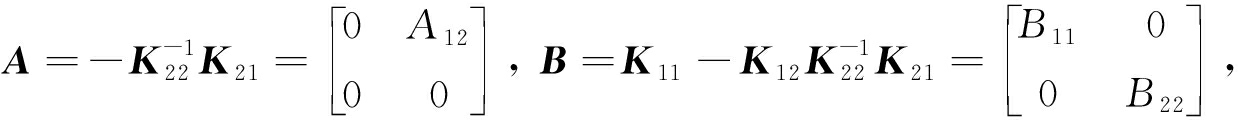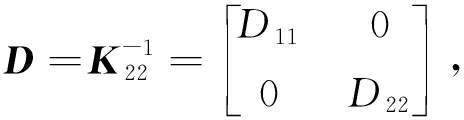A 12 =- K s J 2 /[ K s J 2 -( 2 - φK - K w -i ) ξ 2 ]， B 11 =- 2 + φK + K w +i

B 22 =- K s J 2 ( 2 - φK - K w -i ) ξ 2 /[ K s J 2 -( 2 - φK - K w -i ) ξ 2 ]- I 2 ω 2 D 11 =1/[ K s J 2 -( 2 - φK - K w -i ) ξ 2 ]， D 22 =1/( J 1 - I 2 ω 2 ξ 2 )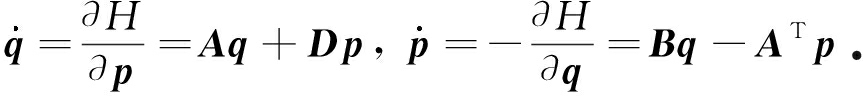(14)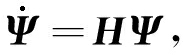(15)

h g Ψ = 0 , when x =0, a ,

(16)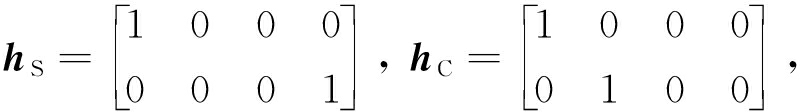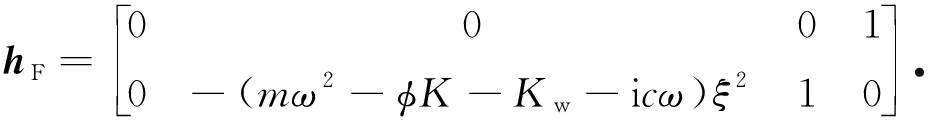## 5 辛本征值和本征解

j = μ j ψ j

(17)

μ 4 - γμ 2 + ζ =0，

(18)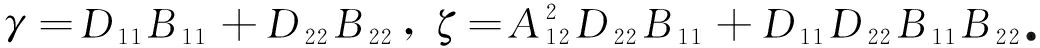μ 1,2 =∓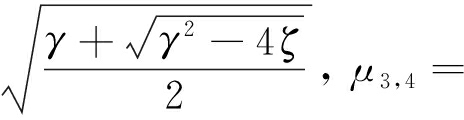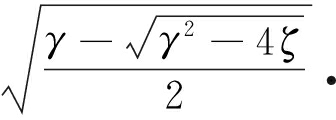(19)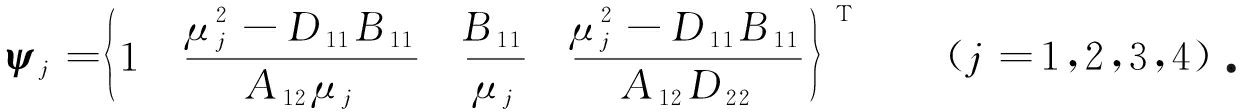(20)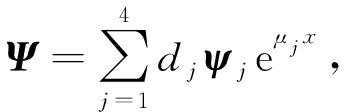(21)

## 6 自 由 振 动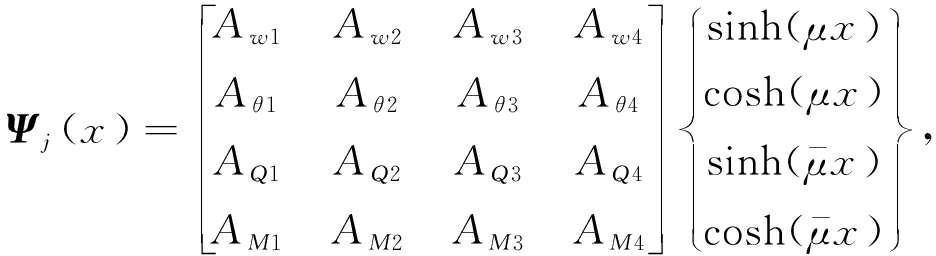(22)

A θj = κ 11 A wn A Mj = κ 12 A wj A Qj = κ 13 A wn ( j =1,2; n =2,1),

(23a)

A θj = κ 21 A wn A Mj = κ 22 A wj A Qj = κ 23 A wn ( j =3,4; n =4,3),

(23b)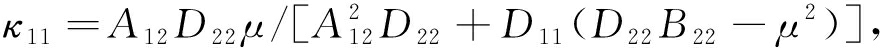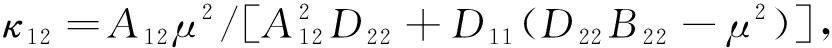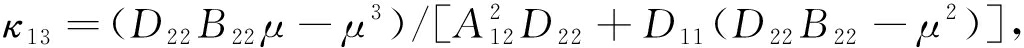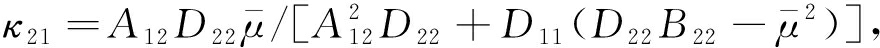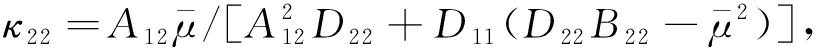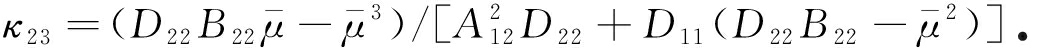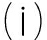SS

ZA w = 0 ,

(24)

f 1 ( ω )=0,

(25)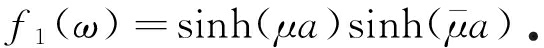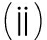CC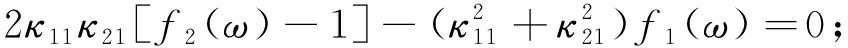(26)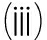CS

κ 11 f 3 ( ω )- κ 21 f 4 ( ω )=0;

(27)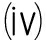CF

κ 21 κ 12 κ 14 + κ 22 κ 11 κ 24 +( κ 11 κ 22 κ 14 + κ 21 κ 12 κ 24 ) f 1 ( ω )-

( κ 11 κ 12 κ 24 + κ 21 κ 22 κ 14 ) f 2 ( ω )=0;

(28)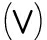SF

κ 12 κ 24 f 4 ( ω )- κ 22 κ 14 f 3 ( ω )=0;

(29)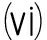FF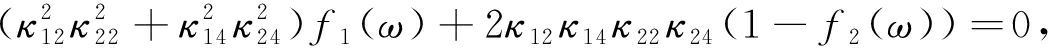(30)

κ 14 = κ 13 + μ ( 2 - φK - K w -i ) ξ 2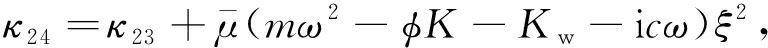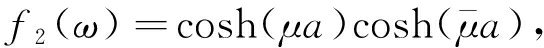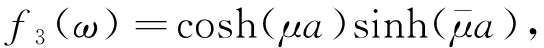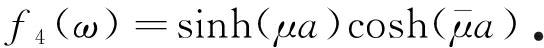## 7 数 值 算 例

### 7 . 1 算例1

E 1 =390 GPa， E 2 =210 GPa， ρ 1 =3 960 kg/m 3 ρ 2 =7 800 kg/m 3

ν 1 = 0.24， ν 2 = 0.30， K s =5/6

b =1 nm， h =1 nm， ρ =1 kg/m 3 E =30 MPa， K s =5/6，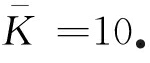Table 1 Fundamental frequencies of an FG nanobeam with 2 ends simply supported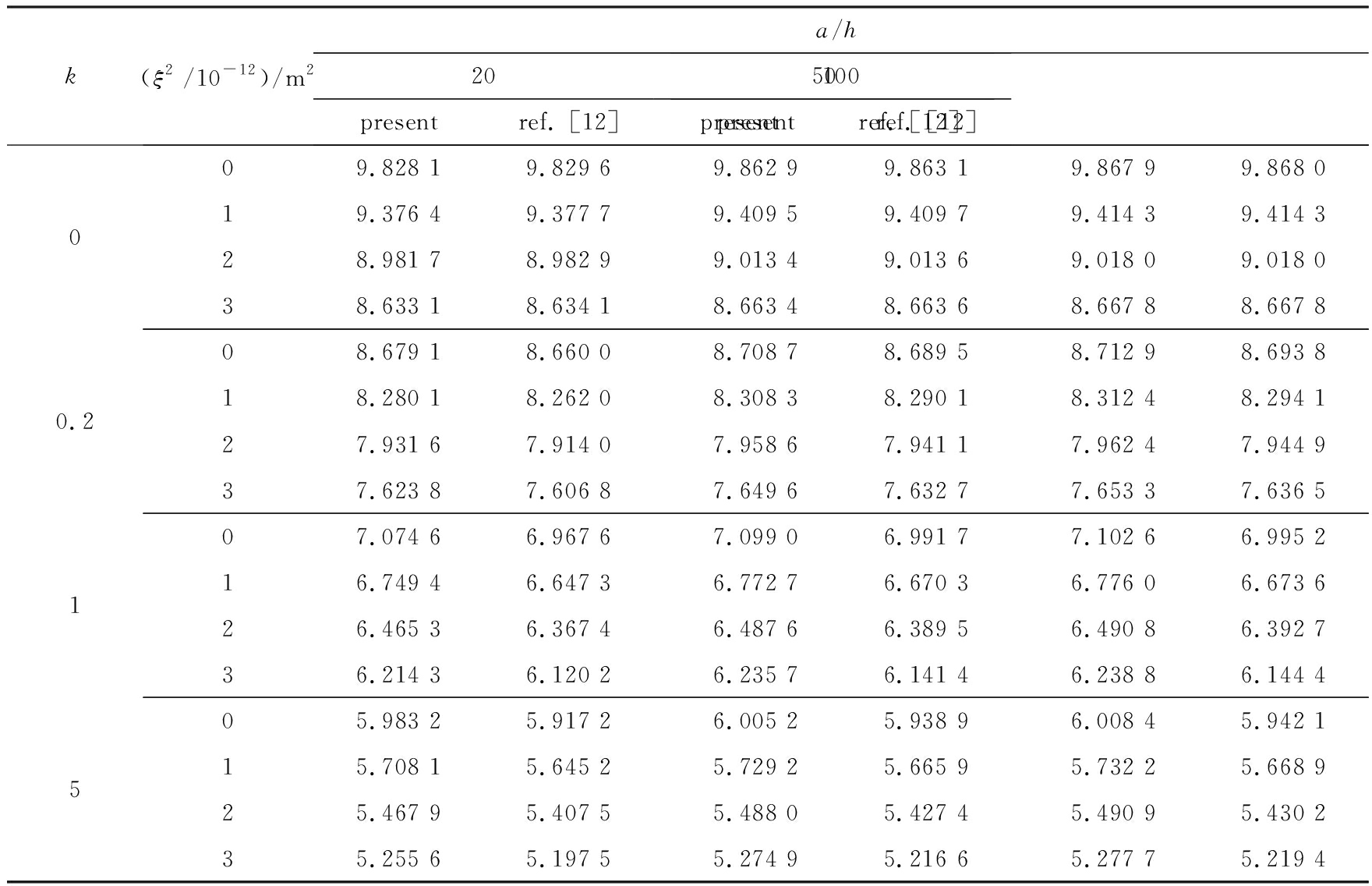Table 2 First 6 in-phase natural frequencies of a double-nanobeam system with 2 ends simply supported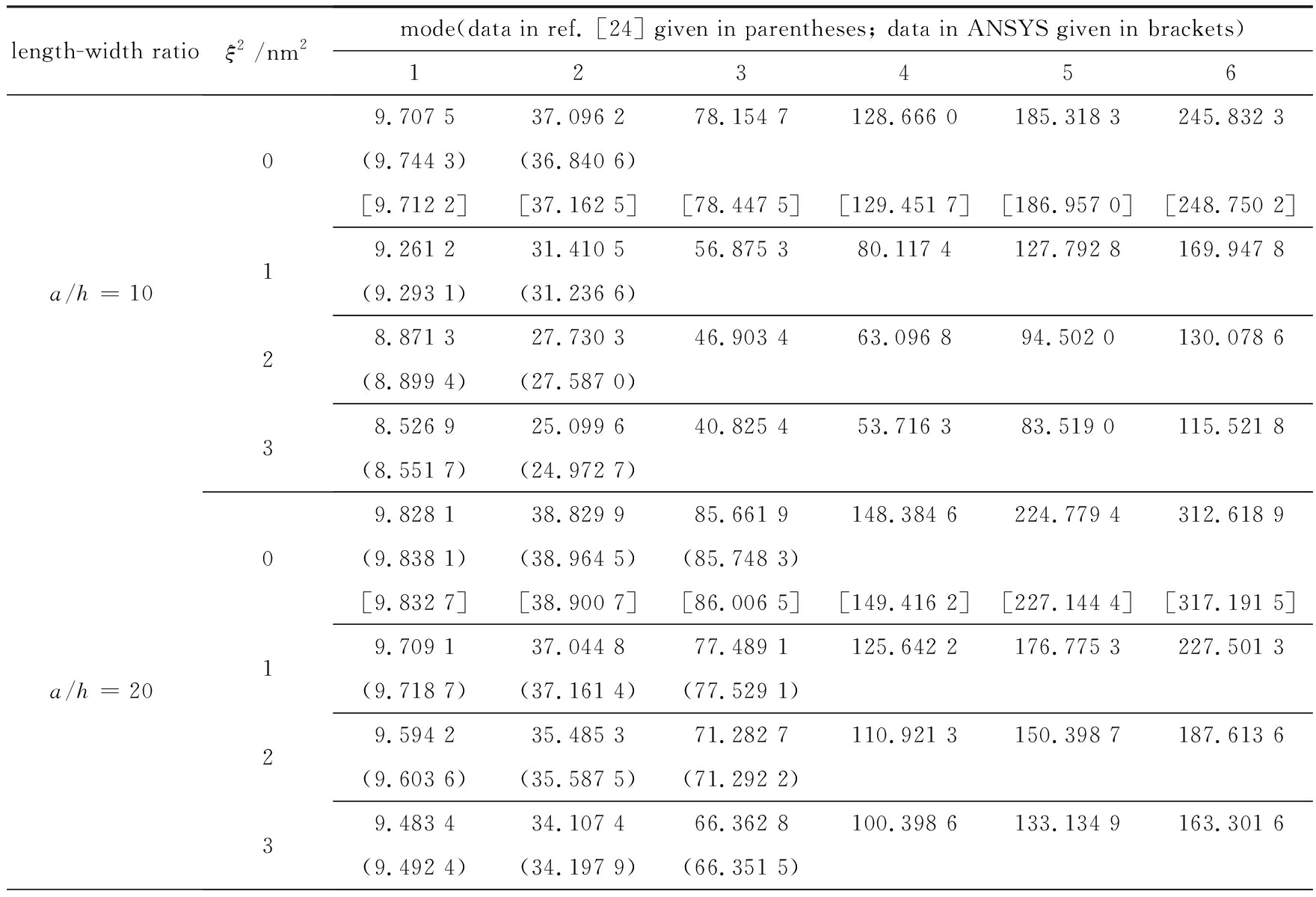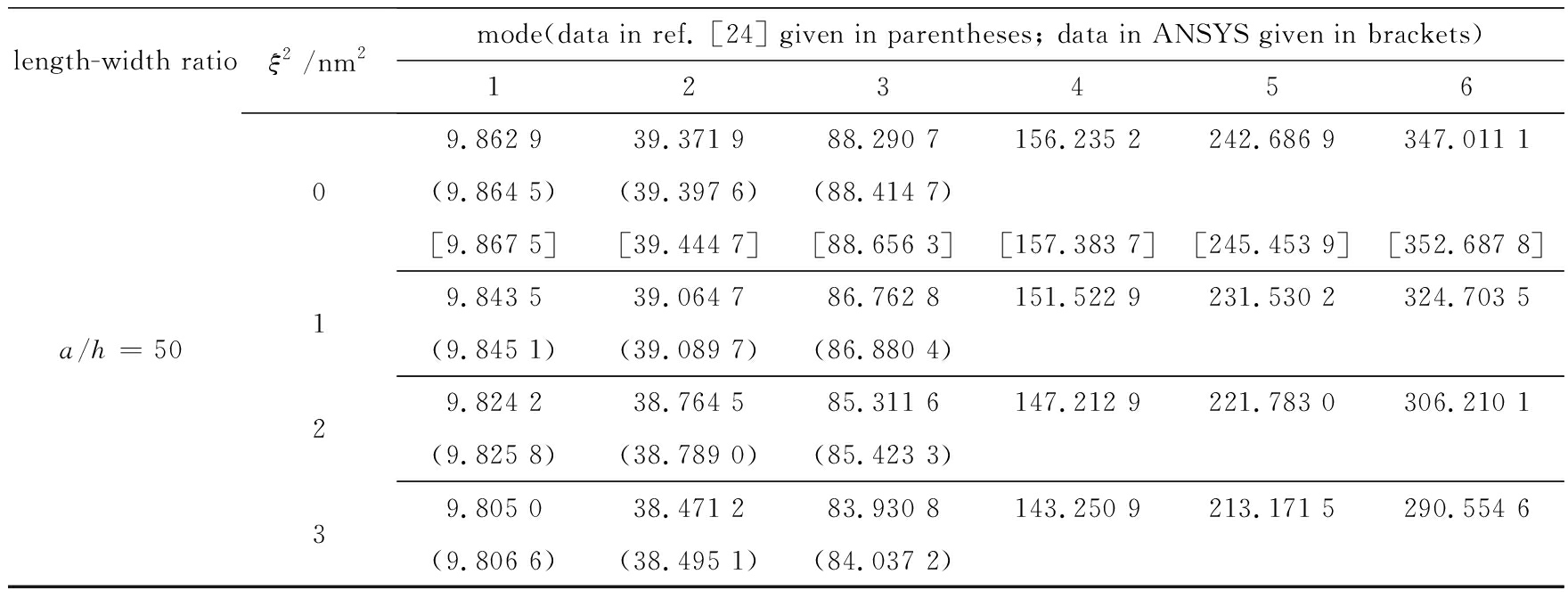### 7 . 2 算例2

Table 3 First 4 in-phase natural frequencies of a functionally graded double-nanobeam

system with various end conditions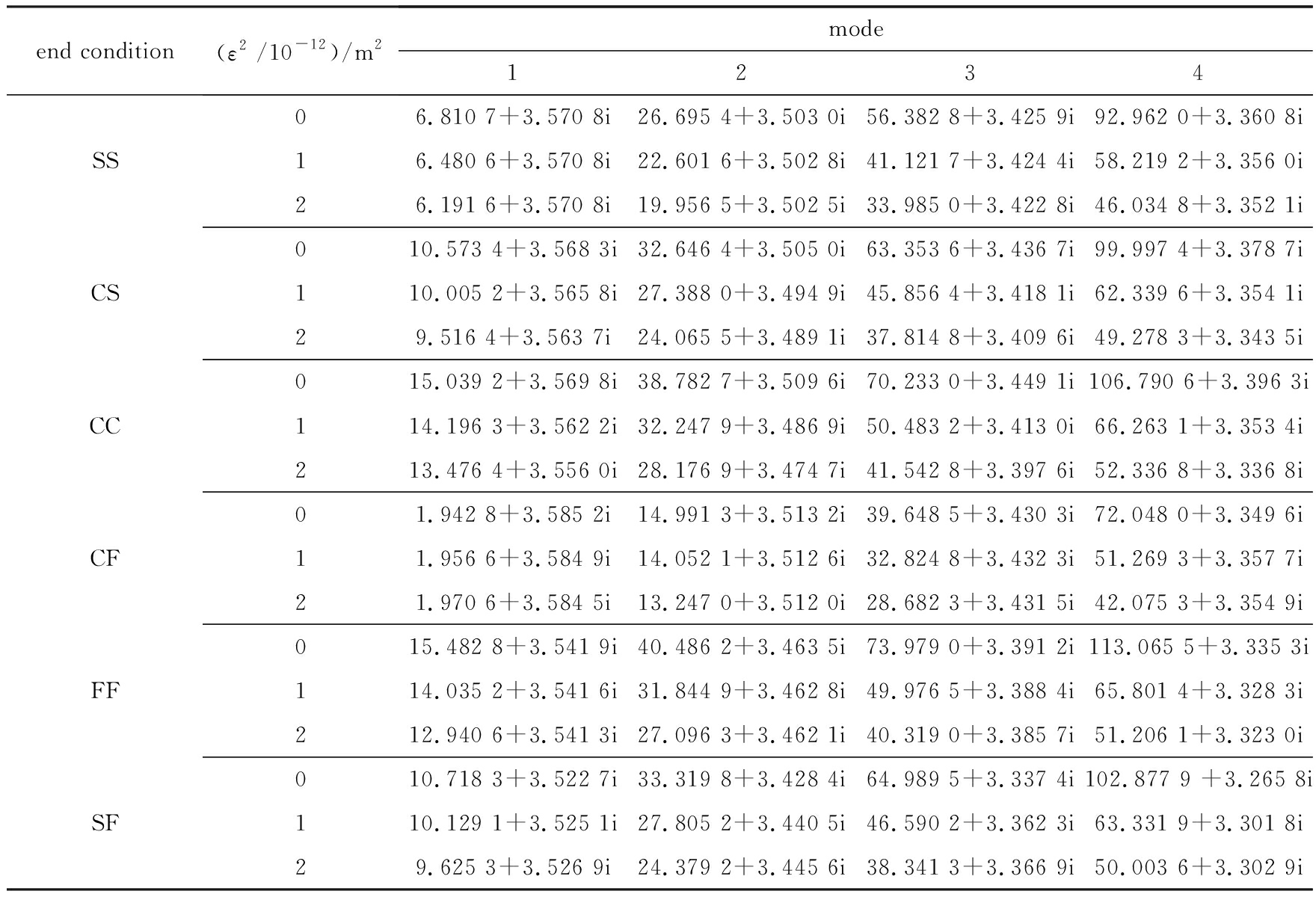Table 4 First 4 out-of-phase natural frequencies of a functionally graded double-nanobeam system with various end conditions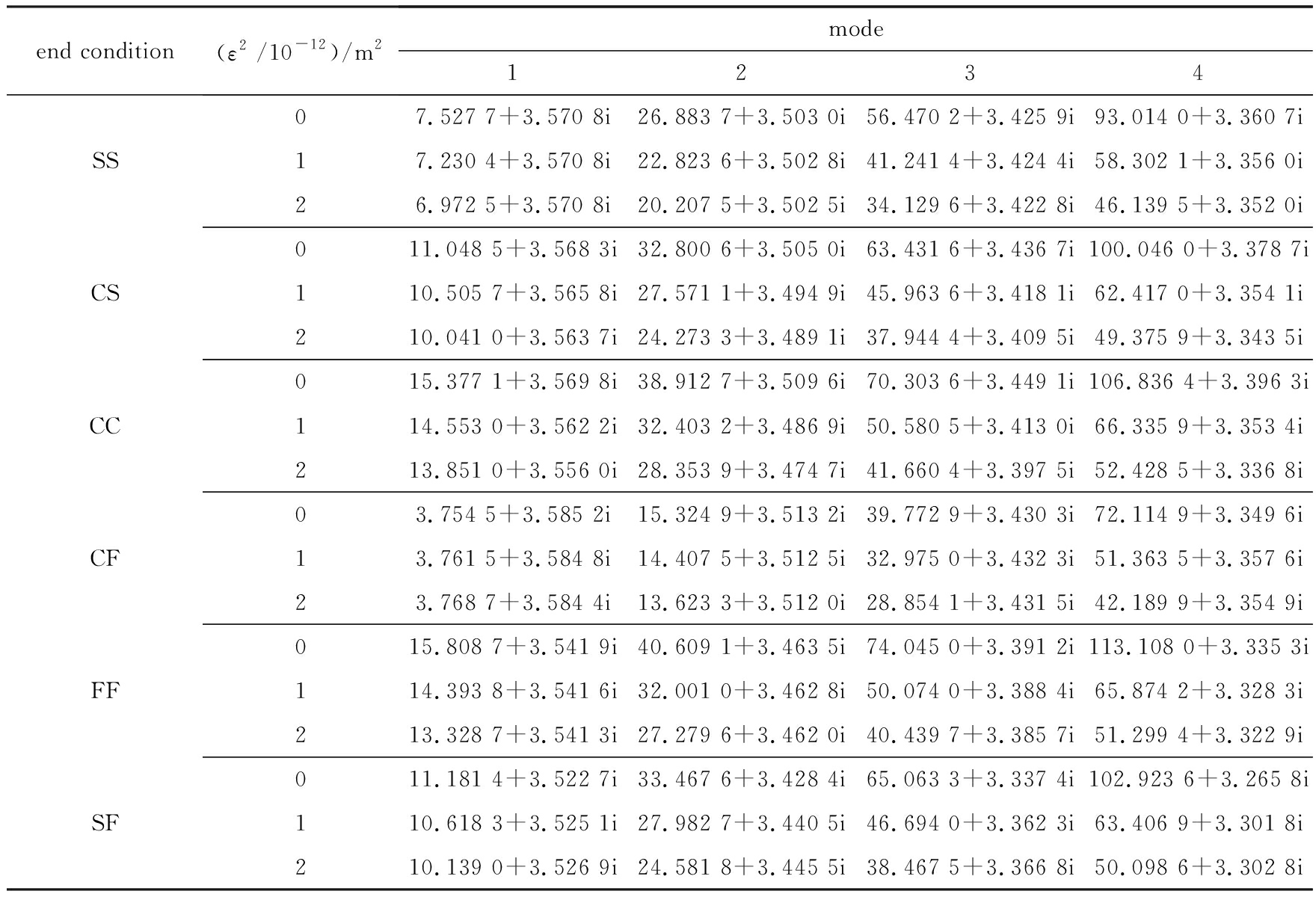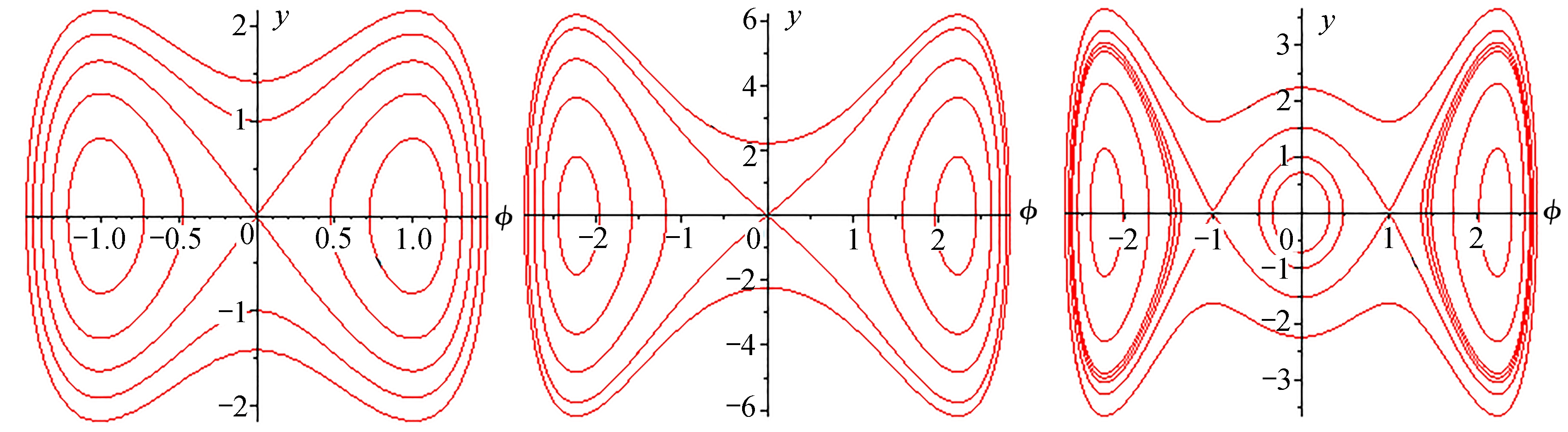(a) 第一阶(b) 第二阶(c) 第三阶(d) 第四阶
(a) The 1st order(b) The 2nd order(c) The 3rd order(d) The 4th order

Fig. 2 First 4 in-phase vibration mode shapes(a) 第一阶(b) 第二阶(c) 第三阶(d) 第四阶
(a) The 1st order(b) The 2nd order(c) The 3rd order(d) The 4th order

Fig. 3 First 4 out-of-phase vibration mode shapes

Table 5 First 4 natural frequencies of an SS functionally graded double-nanobeam system with various power-law indexes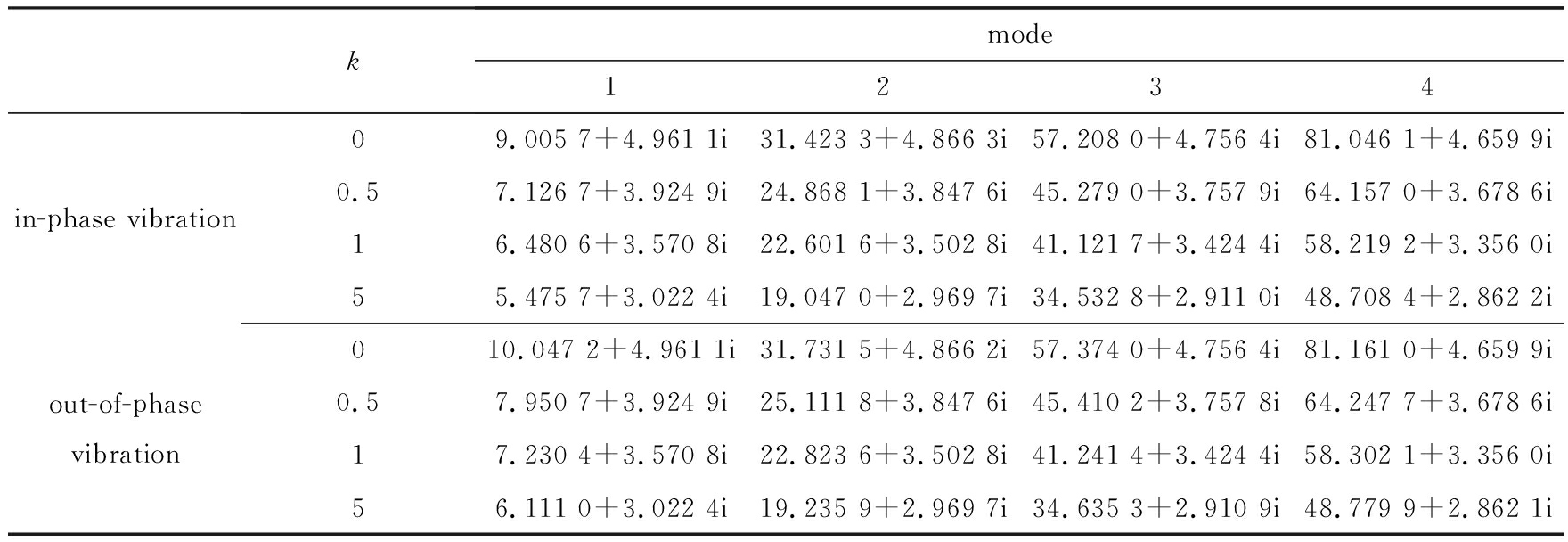Table 6 First 4 natural frequencies of an SS functionally graded double-nanobeam system with various viscoelastic coefficients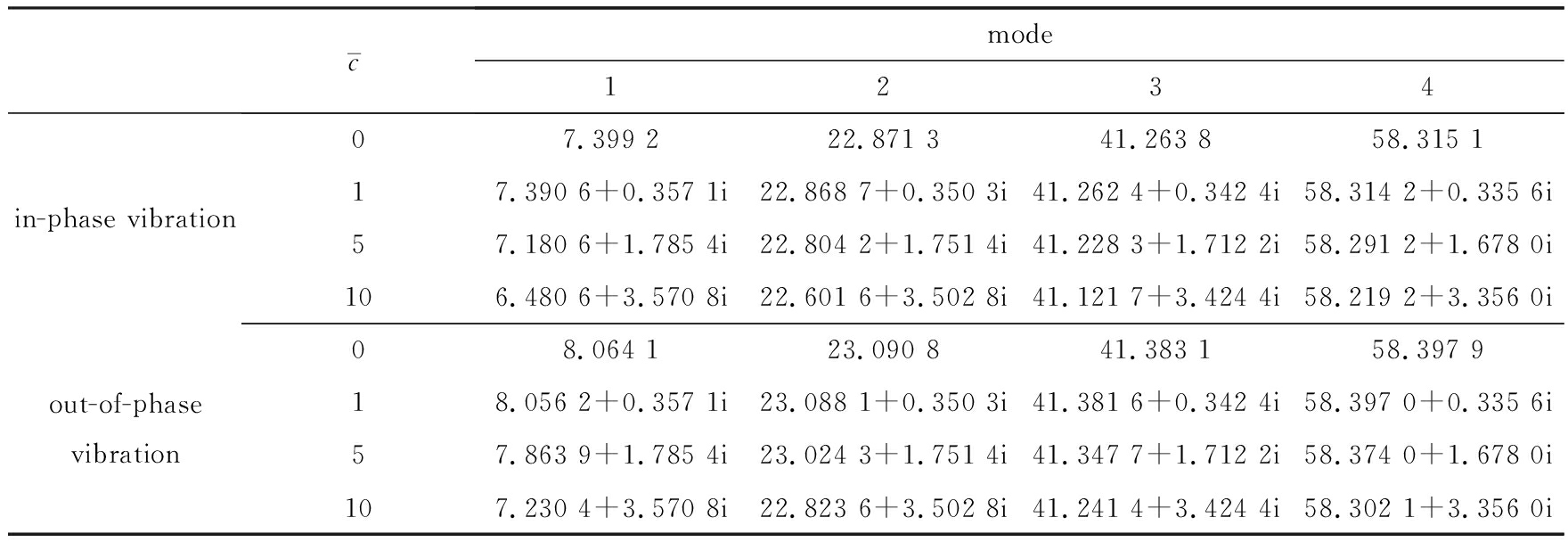## 8 结 论

 EICHENFIELD M, CAMACHO R, CHAN J, et al. A picogram and nanometre-scale photonic-crystal optomechanical cavity[J]. Nature , 2009, 459 (7246): 550-555.

 LIN Q, ROSENBERG J, CHANG D, et al. Coherent mixing of mechanical excitations in nano-optomechanical structures[J]. Nature Photonics , 2010, 4 (4): 236-242

 FU Y, DU H, HUANG W, et al. TiNi-based thin films in MEMS applications: a review[J]. Sensors and Actuators A : Physical , 2004, 112 (2): 395-408.

 KAHROBAIYAN M H, ASGHARI M, RAHAEIFARD M, et al. Investigation of the size-dependent dynamic characteristics of atomic force microscope microcantilevers based on the modified couple stress theory[J]. International Journal of Engineering Science , 2010, 48 (12): 1985-1994.

 ERINGEN A C. On differential-equations of nonlocal elasticity and solutions of screw dislocation and surface-waves[J]. Journal of Applied Physics , 1983, 54 (9): 4703-4710.

 ERINGEN A C. Nonlocal Continuum Field Theories [M]. New York: Springer, 2002.

 RAFII-TABAR H, GHAVANLOO E, FAZELZADEH S A. Nonlocal continuum-based modeling of mechanical characteristics of nanoscopic structures[J]. Physics Reports , 2016, 638 : 1-97.

 林 C W. 基于非局部弹性应力场理论的纳米尺度效应研究: 纳米梁的平衡条件、控制方程以及静态挠度[J]. 应用数学和力学, 2010, 31 (1): 35-50.(LIM C W. On the truth of nanoscale for nanobeams based on nonlocal elastic stress field theory: equilibrium, governing equation and static deflection[J]. Applied Mathematics and Mechanics , 2010, 31 (1): 35-50. (in Chinese))

 尹春松, 杨洋. 考虑非局部剪切效应的碳纳米管弯曲特性研究[J]. 应用数学和力学, 2015, 36 (6): 600-606.(YIN Chunsong, YANG Yang. Shear deformable bending of carbon nanotubes based on a new analytical nonlocal Timoshenko beam model[J]. Applied Mathematics and Mechanics , 2015, 36 (6): 600-606.(in Chinese))

 ELTAHER M A, EMAM S A, MAHMOUD F F. Free vibration analysis of functionally graded size-dependent nanobeams[J]. Applied Mathematics and Computation , 2012, 218 (14): 7406-7420.

 LI L, LI X, HU Y. Free vibration analysis of nonlocal strain gradient beams made of functionally graded material[J]. International Journal of Engineering Science , 2016, 102 : 77-92.

 RAHMANI O, PEDRAM O. Analysis and modeling the size effect on vibration of functionally graded nanobeams based on nonlocal Timoshenko beam theory[J]. International Journal of Engineering Science , 2014, 77 (7): 55-70.

 ELTAHER M A, ALSHORBAGY A E, MAHMOUD F F. Determination of neutral axis position and its effect on natural frequencies of functionally graded macro/nanobeams[J]. Composite Structures , 2013, 99 (5): 193-201.

 NIKNAM H, AGHDAM M M. A semi analytical approach for large amplitude free vibration and buckling of nonlocal FG beams resting on elastic foundation[J]. Composite Structures , 2015, 119 : 452-462.

 EL-BORGI S, FERNANDES R, REDDY J N. Non-local free and forced vibrations of graded nanobeams resting on a non-linear elastic foundation[J]. International Journal of Non - Linear Mechanics , 2015, 77 : 348-363.

 NAZEMNEZHAD R, HOSSEINI-HASHEMI S. Nonlocal nonlinear free vibration of functionally graded nanobeams[J]. Composite Structures , 2014, 110 : 192-199.

 ELTAHER M A, KHAIRY A, SADOUN A M, et al. Static and buckling analysis of functionally graded Timoshenko nanobeams[J]. Applied Mathematics and Computation , 2014, 229 : 283-295.

 LI L, HU Y. Nonlinear bending and free vibration analyses of nonlocal strain gradient beams made of functionally graded material[J]. International Journal of Engineering Science , 2016, 107 : 77-97.

 KE L L, WANG Y S, YANG J, et al. Nonlinear free vibration of size-dependent functionally graded microbeams[J]. International Journal of Engineering Science , 2012, 50 (1): 256-267.

 杨晓东, 林志华. 利用多尺度方法分析基于非局部效应纳米梁的非线性振动[J]. 中国科学: 技术科学, 2010, 40 (2): 152-156.(YANG Xiaodong, LIN Zhihua. The nonlinear vibration of nanoscale beam based on nonlocal effect by multiscale method[J]. Chinese Science : Technical Science , 2010, 40 (2): 152-156. (in Chinese))

 YAO W A, ZHONG W X, LIM C W. Symplectic Elasticity [M]. Hackensack: World Scientific Pubinshing Company, 2009.

 LIM C W, XU X S. Symplectic elasticity: theory and applications[J]. Applied Mechanics Reviews , 2011, 63 (5): 050802.

 ZHOU Z, RONG D, YANG C, et al. Rigorous vibration analysis of double-layered orthotropic nanoplate system[J]. International Journal of Mechanical Sciences , 2017, 123 : 84-93.

 AYDOGDU M. A general nonlocal beam theory: its application to nanobeam bending, buckling and vibration[J]. Physica E : Low - Dimensional Systems and Nanostructures , 2009, 41 (9): 1651-1655.

 ARASH B, WANG Q. A review on the application of nonlocal elastic models in modeling of carbon nanotubes and graphenes[J]. Computational Materials Science , 2012, 51 (1): 303-313.

ZHOU Zhenhuan, LI Yuejie, FAN Junhai, SUI Guohao,ZHANG Junlin, XU Xinsheng

( State Key Laboratory of Structural Analysis for Industrial Equipment ( Dalian University of Technology )； Department of Engineering Mechanics , International Research Center for Computational Mechanics , Dalian University of Technology , Dalian , Liaoning 116024, P . R . China )

Abstract: A new analytical approach was proposed for free vibration of functionally graded (FG) double-nanobeam systems (DNBSs) embedded in viscoelastic medium under the framework of symplectic mechanics and the nonlocal Timoshenko beam theory. In the Hamiltonian system, the dual variables of the displacement and the rotation angle are the generalized shear force and bending moment, respectively. The high-order governing partial differential equations in the classical Lagrangian system were simplified into a set of ordinary differential equations through introduction of an unknown vector composed of the fundamental variables and their dual variables. The free vibration of DNBSs was finally reduced to an eigenproblem in the symplectic space. Analytical frequency equations and vibration mode functions were directly obtained with the symplectic eigensolutions and boundary conditions. Numerical results verify the accuracy and efficiency of the presented method. A systematic parametric study on the small size effect, the interaction between the double nanobeams and the viscoelastic foundation influence, was also provided.

Key words: Hamiltonian system; symplectic method; functionally graded double-nanobeam system; free vibration; analytical solution

Foundation item: The National Natural Science Foundation of China(11672054); The National Basic Research Program of China(973 Program)(2014CB046803); The National Key R&D Program of China(2016YFB0201600)

ⓒ 应用数学和力学编委会，ISSN 1000-0887

DOI: 10.21656/1000-0887.390130